Mathematics
Conics in Real Life
8.6k views

 1 Introduction 2 What Is Conic Section 3 Parabola In Real Life 4 Ellipses In Real Life 5 Hyperbolas In Real Life 6 Summary 7 FAQs

27 October 2020

## Introduction

• Conics or conic sections were studied by Greek mathematicians, with Apollonius of Pergo’s work on their properties around 200 B.C.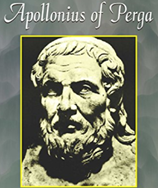• Conics sections are planes, cut at varied angles from a cone. The shapes vary according to the angle at which it is cut from the cone.

• As they are cut from cones, they are called Conies. Further, they have some common properties as they all belong to cones. These curved sections are related to.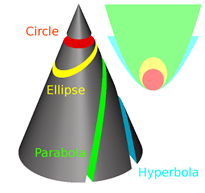## What is Conic Section?

• Conic section is a curve obtained by the intersection of the surface of a cone with a plane.

• In Analytical Geometry, a conic is defined as a plane algebraic curve of degree 2. That is, it consists of a set of points which satisfy a quadratic equation in two variables. This quadratic equation may be written in matrix form. By this, some geometric properties can be studied as algebraic conditions.

• Thus, by cutting and taking different slices(planes) at different angles to the edge of a cone, we can create a circle, an ellipse, a parabola, or a hyperbola, as given below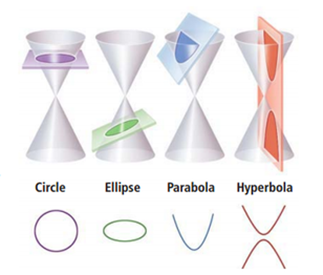• The circle is a type of ellipse, the other sections are non-circular. So, the circle is of fourth type.

Focus, Directrix and Eccentricity

The curve is also defined by using a point(focus) and a straight line (Directrix).

If we measure and let

a – the perpendicular distance from the focus to a point P on the curve,

and b – the distance from the directrix to the point P,

then a: b will always be constant.

$$a: b < 1$$ for ellipse

$$a: b= 1$$ for parabola as $$a= b$$

and $$a: b> 1$$ for hyperbola.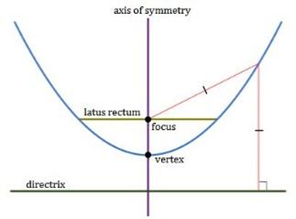Eccentricity: The above ratio a: b is the eccentricity.

Thus, any conic section has all the points on it such that the distance between the points to the focus is equal to the eccentricity times that of the directrix.

Thus, if eccentricity $$<1$$, it is an ellipse.

if eccentricity $$=1$$, it is a parabola.

and if eccentricity $$=1$$, it is a hyperbola.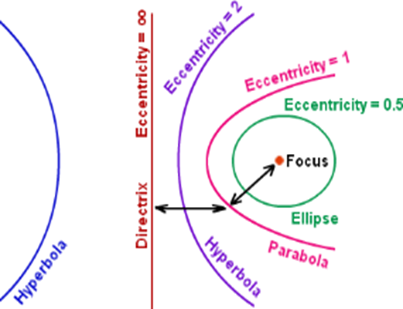For a circle, eccentricity is zero. With higher eccentricity, the conic is less curved.

### Latus Rectum

The line parallel to the directrix and passing through the focus is Latus Rectum.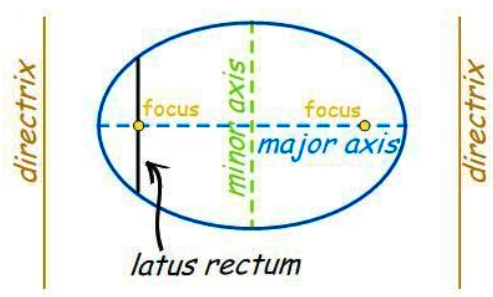Source: i2.wp.com/c1.staticflickr.com

Length of Latus Rectum = 4 times the focal length

Length $$=\frac{2b^2}{a}$$ where $$a =\frac{1}{2}$$ the major diameter

and $$b =\frac{1}{2}$$ the minor diameter.

= the diameter of the circle.

Ellipse has a focus and directrix on each side i.e., a pair of them.

### Equations

General equation for all conics is with cartesian coordinates x and y and has $$x^2$$ and $$y^2$$ as

the section is curved. Further, x, y, x y and factors for these and a constant is involved.

Thus, the general equation for a conic is

$Ax^2 + B x y + C y^2+ D x + E y + F = 0$

Using this equation, following equations are obtained:

For ellipse, $$x^2a^2+y^2b^2=1$$

For hyperbola, $$x^2a^2-y^2b^2=1$$

For circle, $$x^2a^2+y^2a^2=1$$ (as radius is a)

## Parabola in Real Life

• Parabola is obtained by slicing a cone parallel to the edge of the cone. It is of U – shape as a stretched geometric plane. This formula is $$y =x^2$$ on the x – y axis.

• Mathematician Menaechmus derived this formula.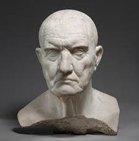• Parabola is found in nature and in works of man.

• Water from a fountain takes a path of parabola to fall on the earth.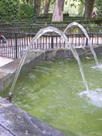• A ball thrown high, follows a parabolic path.

• A roller coaster takes the path of rise and fall of a parabolic track of the sea.

• An architectural structure built and named The Parabola in London in 1962 has a copper roof with parabolic and hyperbolic linings.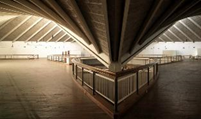• The Golden Gate Bridge in San Francisco in California is famous with parabolic spans on both sides.• In light houses, parabolic bulbs are provided to have a good focus of beam to be seen from distance by mariners.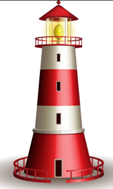• Automobile headlights are also with parabola type.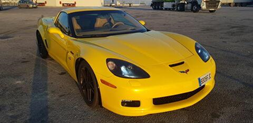• The stretched arc of a rocket launch is parabolic.• The satellite dish is a parabolic structure facilitating focus and reflection of radio waves.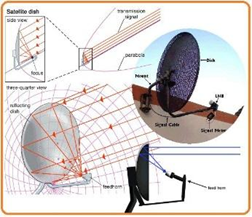## Ellipses in Real Life

• Electrons in the atom move around the nucleus in an elliptical path of orbit.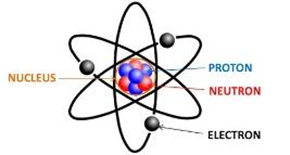• Property of Ellipse to reflect sound and light is used in pulverizing kidney stones. The patient is laid in an elliptical tank of water. Kidney stones being at the other focus are concentrated and pulverized.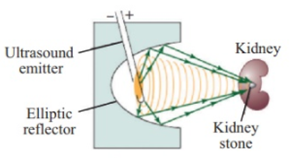• Paul’s Cathedral is an elliptical shaped structure to facilitate talking at one end is heard at the other end using the property of ellipse.

• There is an ellipse shaped park in front of White House in Washington.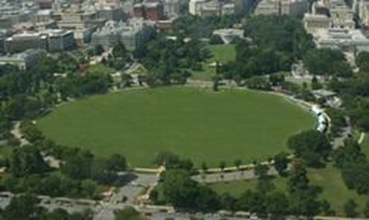• When a tumbler of water is tilted, an elliptical surface of water is seen.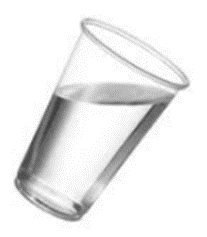• Food items carrot, cucumber cut at an angle to its main axis results in elliptical shape and elegant look.• Whispering galleries at US Statutory capital and St. Paul’s Cathedral, London demonstrates the property of the ellipse that one’s whisper from one focus can be heard at the other focus by only a person to whom it is sent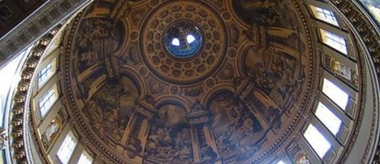• Elliptical training machines enable running or walking without straining the heart.## Hyperbolas in Real Life

• A guitar is an example of hyperbola as its sides form hyperbola.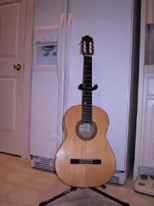• Dulles Airport has a design of hyperbolic parabolic. It has one cross-section of a hyperbola and the other a parabola.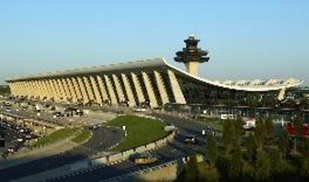• Gear Transmission having pair of hyperbolic gears. It is with skewed axles and hourglass shape giving hyperbola shape. The hyperbolic gears transmit motion to the skewed axle.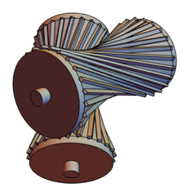• The Kobe Port Tower has hourglass shape, that means it has two hyperbolas. Things seen from a point on one side will be the same when seen from the same point on the other side.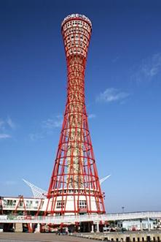• Satellite systems, Radio systems use hyperbolic functions.

• Inverse relationship is related to hyperbola. Pressure and Volume of gas are in inverse relationships. This can be described by a hyperbola.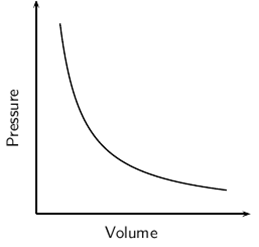• Lens, monitors, and optical glasses are of hyperbola shape.

## Summary

Conic or conical shapes are planes cut through a cone. Based on the angle of intersection, different conics are obtained. Parabola, Ellipse, and Hyperbola are conics. Circle is a special conic. Conical shapes are two dimensional, shown on the x, y axis. Conic shapes are widely seen in nature and in man-made works and structures. They are beneficially used in electronics, architecture, food and bakery and automobile and medical fields.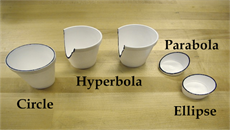## What are the 4 types of conic sections?

According to the angle of intersection between a plane and a cone, four different conic sections are obtained. They are Parabola, Ellipse, Hyperbola, and Circle. They are two dimensional on the x-y axis.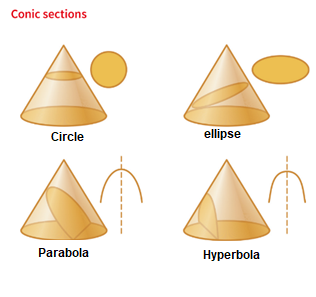## How do we obtain Conic Sections?

Conic section involves a cutting plane, surface of a double cone in hourglass form and the intersection of the cone by the plane. According to the angle of cutting, that is, light angle, parallel to the edge and deep angle, ellipse, parabola and hyperbola respectively are obtained. Circle is also conic, and it is cut parallel to the circular bottom face of the cone.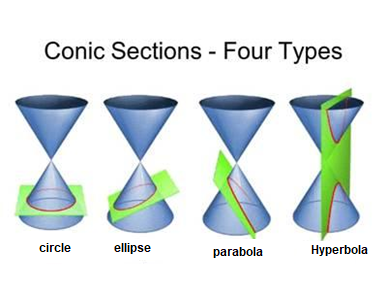## How do you find the area of an ellipse?

Area of an ellipse is $$(a \times b \times π)$$ sq. units.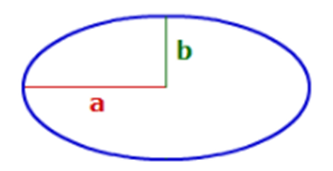where a = length of major axis of ellipse

b = length of minor axis of ellipse.

## What are some real-life applications of conics?

Planets travel around the Sun in elliptical routes at one focus.

Mirrors used to direct light beams at the focus of the parabola are parabolic.

Parabolic mirrors in solar ovens focus light beams for heating.

Sound waves are focused by parabolic microphones.

Car headlights and spotlights are designed based on parabola’s principles.

The path travelled by objects thrown into air is parabolic.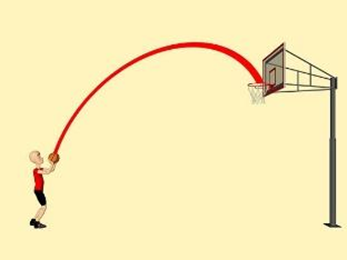Hyperbolas are used in long range navigation systems called LORAN.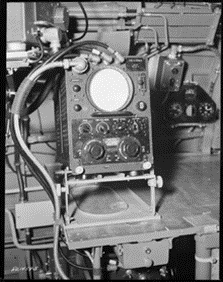Telescopes use parabolic mirrors.